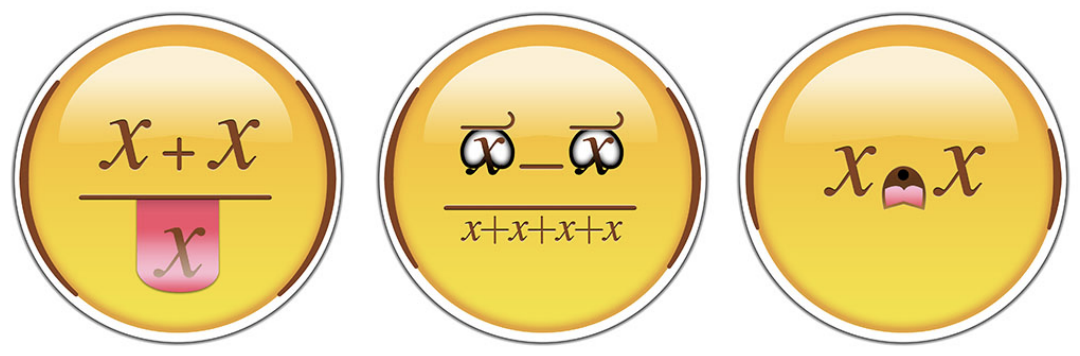시간 제한메모리 제한제출정답맞힌 사람정답 비율
4 초 (추가 시간 없음) 512 MB84350.000%

## 문제Here’s a cool puzzle: Can you write a mathematical expression that evaluates to 113, but only contains common mathematical symbols and four instances of the digit 4?

One possible answer is the following:

$113 = \frac{\sqrt{4} + (\sqrt{4} + 4!)%}{\sqrt{4}%}$

Here, ! is the factorial symbol, and % is the percentage symbol, e.g., 11% = 0.11.

Four fours is an arithmetic puzzle/exercise where the goal is to produce a given number using only four fours and common mathematical symbols. For some numbers, this is easy, e.g. 16 can be written as 4 + 4 + 4 + 4. But for others, it isn’t always so, as you may have seen from the example 113 above. As another example, can you produce 2016 using only four fours?

In this problem, you will solve a variation of this puzzle. Given x and y, find a valid mathematical expression that evaluates to y, subject to the following constraints:

• The only constant appearing in the expression must be the integer x.
• The only operations allowed are addition, subtraction, multiplication and division.
• The number of operations must be at most 28.
• Intermediate results cannot exceed 1018 in absolute value.
• Division must be valid and must always yield integers.

If no such expression exists, output IMPOSSIBLE.

## 입력

The first line of input will contain an integer T, the number of test cases.

The first and only line of each test case will contain two space-separated integers, x and y, as discussed in the problem statement.

Constraints

• 1 ≤ T ≤ 212121
• 0 ≤ x, y < 1212

## 출력

For each test case output a single line containing a single string.

• If no such expression exists, output the string IMPOSSIBLE.
• Otherwise, output a string containing only the characters x, + -, *, or /. This represents your expression written in postfix notation.

An expression in postfix notation is evaluated with a stack, initially empty. Then, for each letter from left to right:

• If it is x, then the number x is pushed onto the stack.
• If it is +, then two numbers b and a (in that order) are popped from the stack, and then a+b is pushed onto the stack.
• If it is -, then two numbers b and a (in that order) are popped from the stack, and then a−b is pushed onto the stack.
• If it is *, then two numbers b and a (in that order) are popped from the stack, and then a×b is pushed onto the stack.
• If it is /, then two numbers b and a (in that order) are popped from the stack, and then a/b is pushed onto the stack.

At the end of this process, the stack must contain exactly one number, which we define as the result of the evaluation. If the stack doesn’t contain exactly one number, or if an empty stack is popped, then the evaluation fails.

There may be multiple correct answers; any one will be accepted.

## 예제 입력 1

4
4 32
3 21
3 21
3 21


## 예제 출력 1

xx*xx*+
xxxx+*+
xxxx+*+
xxx**x-x-